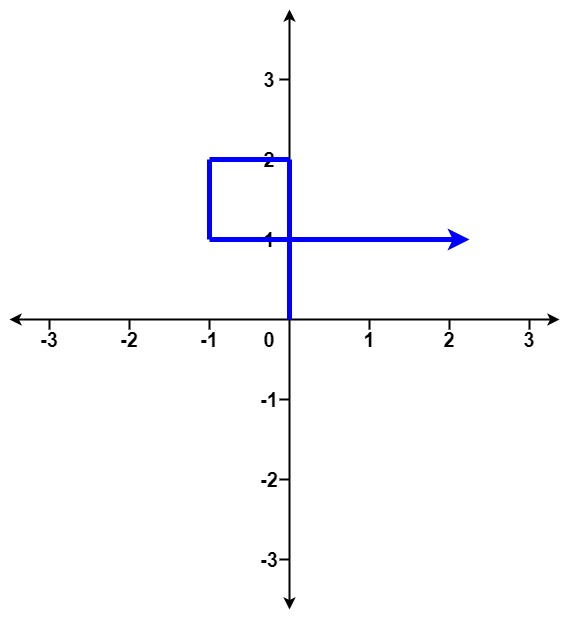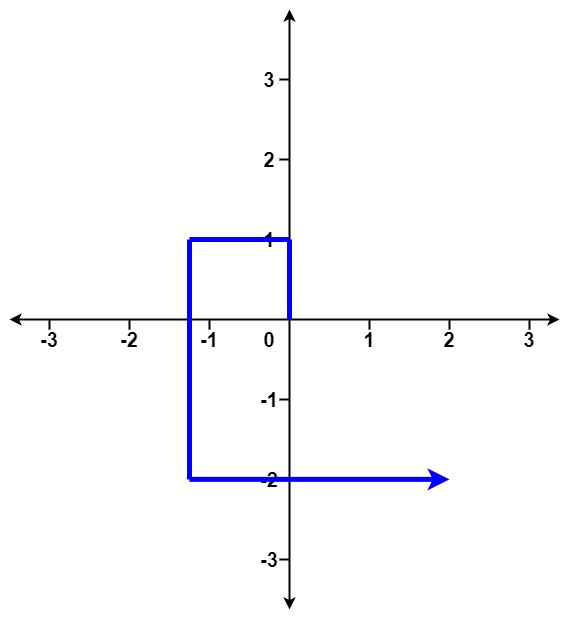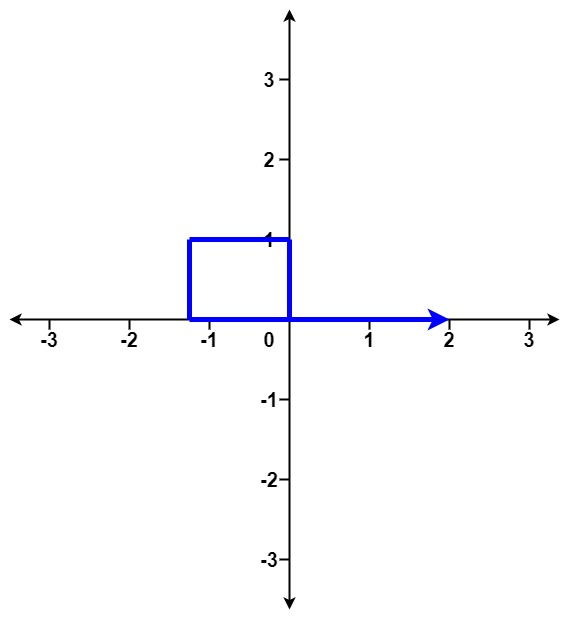# GeetCode Hub

You are given an array of integers `distance`.

You start at point `(0,0)` on an X-Y plane and you move `distance` meters to the north, then `distance` meters to the west, `distance` meters to the south, `distance` meters to the east, and so on. In other words, after each move, your direction changes counter-clockwise.

Return `true` if your path crosses itself, and `false` if it does not.

Example 1:```Input: distance = [2,1,1,2]
Output: true
```

Example 2:```Input: distance = [1,2,3,4]
Output: false
```

Example 3:```Input: distance = [1,1,1,1]
Output: true
```

Constraints:

• `1 <= distance.length <= 105`
• `1 <= distance[i] <= 105`

class Solution { public boolean isSelfCrossing(int[] distance) { } }